## Friday, September 14, 2012

### The Colored Orbit Buddhabrot

In this post, I'll discuss what I believe to be a new method for coloring the Buddhabrot, which was conceived of by my brother, Michal Dichter, and that I coded in MATLAB.

Background

The Buddhabrot is a fractal derived from the Mandelbrot set, which is also a fractal. If you'd like to first learn what the Buddhabrot is, you can do so
here or here.

Ordinarily, a Buddhabrot is generated by iterating a large number of randomly chosen complex points according to the Mandelbrot equation and only considering orbit lengths between 1 and 100, for example. This method would yield an image like this.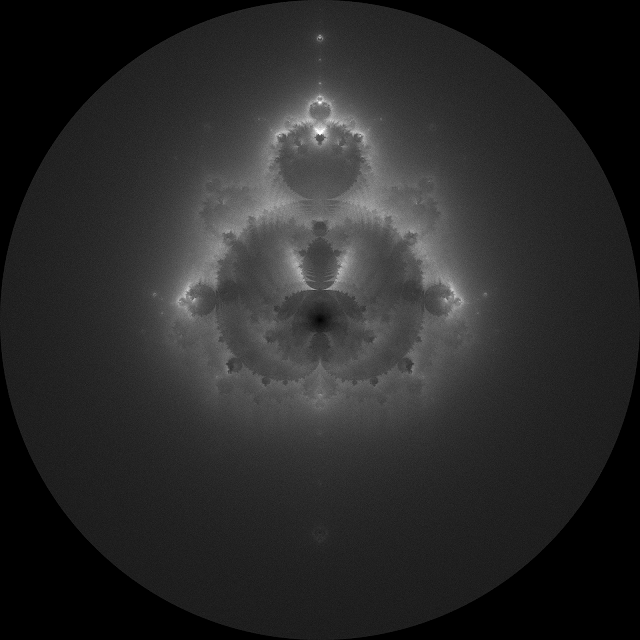97,000,000 orbits of lengths between 1 and 100. Highlights boosted in Photoshop.
It is known that orbit lengths on the order of roughly 20,000 or greater yield much more visually interesting orbits because they linger for many more iterations. However, these orbits are exceptionally difficult to find, as their initial points invariably lie just outside the border of the Mandelbrot set. Even with more sophisticated orbit finding techniques, it may be difficult to find more than a few thousand such orbits in one day of computational time.

Consequently, in the same amount of computational time, fewer orbits are found, and as a result, the Buddhabrot is less dominated by random noise (above) and individual orbits can clearly be seen (below).268 orbits of lengths between 100,000 and 250,000. 3rd root taken (explained later).
The Colored Orbit Method

When plotting the Buddhabrot conventionally, no distinction is made between individual orbits. They are all summed together to form the final Buddhabrot image.

In this method, each orbit is assigned a random RGB color and then added to the final Buddhabrot image. Here is some output using this method: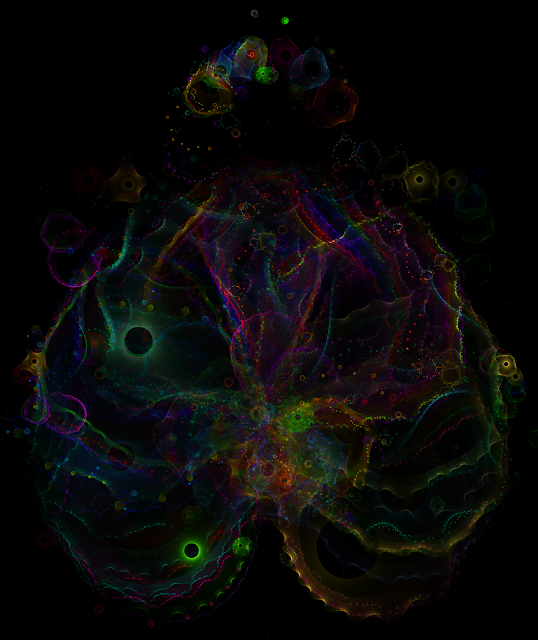268 randomly colorized orbits of lengths between 100,000 and 250,000. (cropped, highlights boosted) There are plenty of post-processing techniques that could be applied to this image to increase its aesthetic appeal, including adjusting the saturation, shifting hues, and selective blurring. For the image above, I shifted the hues and boosted the highlights slightly. Coding implementation In my code, I start by loading a matrix of initial points (top row) and their orbits lengths (bottom row), which, in this case, are between 100,000 and 250,000. How I found these orbits is a blog post in and of itself, so for now, let's just take them for granted. Here are the first three columns.

-0.6388 + 0.3730i
-0.3710 - 0.5960i
-0.3485 - 0.6056i
178393
185217
157494

Each initial point is iterated normally, according to the Mandelbrot equation, and a temporary Buddhabrot matrix is created for just that one orbit. Here's what this matrix looks like for a single ordinary orbit.Orbit of the point -0.3485 - 0.6056i. Orbit length = 157,494. (detail)
My code randomly assigns an RGB value to each orbit, normalizes it (explained below), and then adds it to the final Buddhabrot image, similar to how a Nebulabrot is made, but with numerous color channels instead of three. When all the orbits have been added, the code normalizes the image matrix so that none of the color channels exceed 255.

Individually normalizing orbits

For most orbits, the range of the image matrix - the difference between the value of the most-hit pixel and the least-hit pixel - is between 10 and 100. In the image above, for example, the range is 23.

However, there are orbits with extremely high ranges on the order of 750 or greater. These are problematic because when working with grayscale images, the RGB standard only allows for 256 shades of gray. Therefore it is technically impossible to view such orbits as they truly are. We can view an approximation of the orbit by rescaling the matrix to fit the RGB standard by multiplying the entire matrix by 255/max, where max is the highest value in the matrix.

Here is a single orbit with a range of about 1,000. This image was rescaled as described above.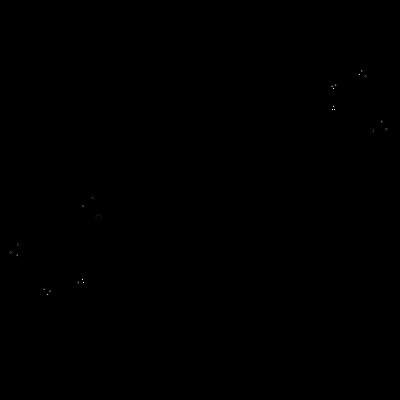Orbit of the point -0.2279 + 0.7457i. Orbit length = 128,435. Range before rescaling = 798. (detail)
High range orbits are tiny. They exist in smaller areas than low range orbits, therefore the total number of hits, the orbit length, must be packed into a smaller area. This causes the number of hits per pixel to be relatively high.

It is usually true that when Buddhabrot matrices have extremely high ranges, the frequency of each value between 0 and max appearing in the matrix is not uniform over the entire range. In other words, a handful of pixels are so exceptionally bright that they reduce darker regions to pure blacks when the image is rescaled.

To make an analogy, if you look up at the sky on a clear night, you will be able to see some number of bright stars. Yet there are also countless nebulae and dimmer stars you cannot see because the bright stars are so bright that they obscure everything else. In this case, trying to theoretically divide the perceived brightness by some number would be of no use in seeing the fainter objects. To do this, the proportionality between the light and dark regions must itself be altered.

The solution is taking the nth root of the entire matrix. This is a fairly standard data transformation. Consider the shape of the function y=sqrt(x), plotted here against y=x for comparison.

This function alters values more intelligently than simply dividing or multiplying them by a constant. The higher a value, the more it is downscaled. Meanwhile, lower values remain about the same (relatively speaking).

It is important to realize that this transformation reduces the range of the image. For example, taking the 3rd root reduces the range more than would taking the 2nd root. In my code, I determine which root, n, is necessary to reduce the range to a pre-set value,
rangeSet. Here's the math behind this:

$\text{range}^{1/n} = \text{rangeSet}$ $1/n = \text{log}_{\text{range}} \text{rangeSet}$ $1/n = \frac{\text{log(rangeSet)}}{\text{log(range)}}$ $n = \frac{\text{log(range)}}{\text{log(rangeSet)}}$

Setting rangeSet equal to 255 would be logical. However, the more the range is reduced, the more of the orbit becomes visible. The tradeoff, though, is that there are fewer shades of gray in the resulting image. A good value for rangeSet, then, should expose as much of the orbit as possible while preserving enough shades of gray that the image appears smooth. I have found that a value of 25 works well. Here's the orbit shown earlier, before and after the transformation.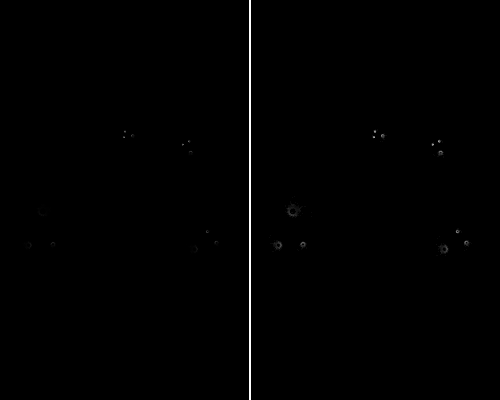Orbit of the point -0.2279 + 0.7457i before and after root transformation. (detail)
While the difference is not dramatic in this example, it's important to perform this transformation to intelligently equalize the relative weight of the individual orbits before they are added to the final image. Aesthetically, it also produces smoother-looking orbits by providing gradience around bright pixels.

Gallery

Here are some of the images I have generated with the techniques described above.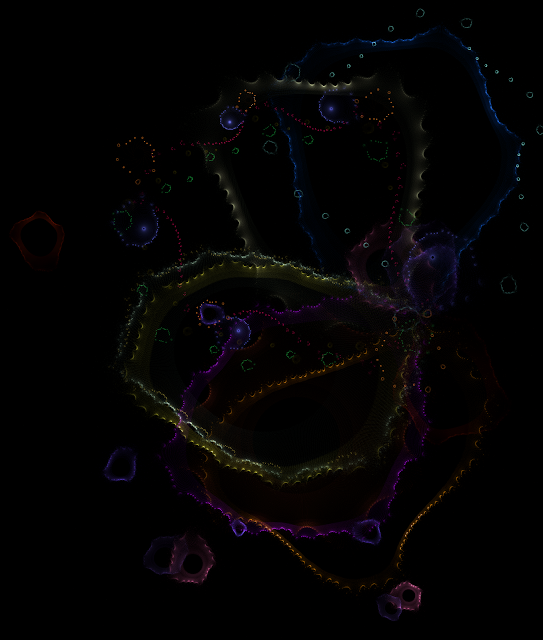A few colorized orbits of lengths between 100,000 and 250,000. Highlights boosted in Photoshop. (detail)Approximately 250 orbits of lengths on the order of 100,000. Highlights boosted in Photoshop. (crop)Approximately 250 orbits of lengths on the order of 50,000. Saturated and highlights boosted in Photoshop. (crop)

#### 1 comment:

1.Normally, adding in colors early in the rendering process leads to a problem (as you described). That can be solved with histogram equalization and colorization added after that. You found a decent alternate solution which lets you keep the individually colored orbits. These are some very lovely results, Daniel.